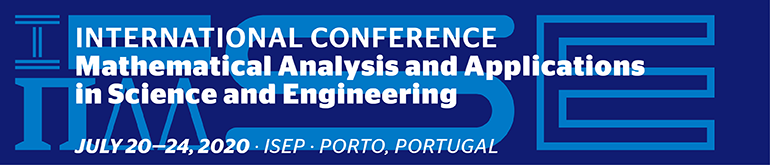Journal Browser

# Conferences

## 20–24 July 2020, Porto, Portugal International Conference on Mathematical Analysis and Applications in Science and Engineering – ICMA2SC'20The conference aims to bring together researchers in every discipline of applied mathematics, science, engineering, industry, and technology, to discuss the development of new mathematical models, theories, and applications that contribute to the advancement of scientific knowledge and practice.

We expect the authors to propose research including topics such as partial and ordinary differential equations, integer and fractional order equations, linear algebra, numerical analysis, operations research, discrete mathematics, optimization, control, probability, computational mathematics, amongst others.

The conference is designed to maximize the involvement of all participants and will present the state-of-the-art research and the latest achievements.

Main topics include:

Ordinary and partial differential equations: theory and applications;

Mathematical modelling involving time fractional ODEs and PDEs;

Integral equations and integral transforms;

Uncertainty quantification in mathematical modelling;

Control theory, optimization and their applications;

Probability, statistics and numerical analysis;

Inverse problems: modelling and simulation;

Computational methods in sciences and engineering;

Fractional dynamic systems and applications;

Fractional signals and systems;

Singularities analysis and integral representations for fractional differential systems;

Special functions related to fractional calculus;

Applications in biological systems and cancer dynamics;

Applications to electrical engineering, electronics, electromagnetism, electrochemistry, finance and economics, fractional earth science, image processing, robotics, automatic control, mechanics, viscoelasticity, thermal engineering;

History of fractional calculus.

https://www.isep.ipp.pt/Page/ViewPage/ICMASC